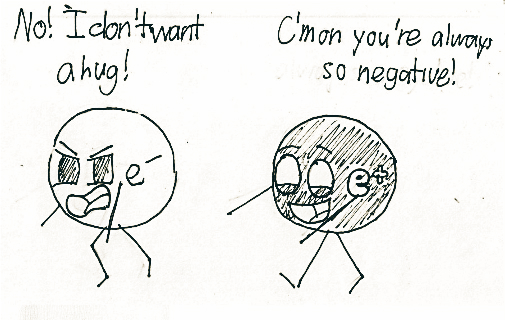# Who remembers to subtract?If $-1.6\times { 10 }^{ -19 }$ is less than $+1.6\times { 10 }^{ -19 }$ by $3.2\times { 10 }^{ -19 }$,

Can we say that the electron has a charge $\displaystyle 3.2\times { 10 }^{ -19 }C$ less than the charge of a proton?

×